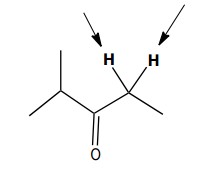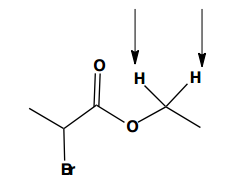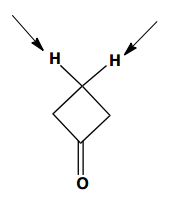Clutch Prep is now a part of Pearson
Ch. 15 - Analytical Techniques: IR, NMR, Mass SpectWorksheetSee all chapters

# 1H NMR: Q-Test

See all sections
Sections
Purpose of Analytical Techniques
Infrared Spectroscopy
Infrared Spectroscopy Table
IR Spect: Drawing Spectra
IR Spect: Extra Practice
NMR Spectroscopy
1H NMR: Number of Signals
1H NMR: Q-Test
1H NMR: E/Z Diastereoisomerism
H NMR Table
1H NMR: Spin-Splitting (N + 1) Rule
1H NMR: Spin-Splitting Simple Tree Diagrams
1H NMR: Spin-Splitting Complex Tree Diagrams
1H NMR: Spin-Splitting Patterns
NMR Integration
NMR Practice
Carbon NMR
Structure Determination without Mass Spect
Mass Spectrometry
Mass Spect: Fragmentation
Mass Spect: Isotopes

Concept #1: Q-Test (Proton Relationships)

Transcript

We just learned an assumption that stated that all of the hydrogen on a single atom are going to share one peak on Proton NMR and while that assumption is very helpful for most cases there are going to be some exceptions where these protons will actually have different relationships depending on the original chirality of the molecule, this is where the exceptions begin and if you learn to accept the exceptions and just roll with them this is going to go a lot smoother for all of us so let's dig right into proton relationships and the Q test.

So, as I just mentioned sometimes these hydrogens are going to have different relationships based on their original chirality and the test that we use for that is called the Q test so what the heck is the Q test? Well the Q test is simply this what we do is let's just look at this example here we have 3 hydrogens we take 1 hydrogen we cross it out and we replace it with a random letter in this case Q the only reason Q is just to be random so that we don't confuse it with an atom, OK? So, we're going to replace H with the symbol Q and we're going to analyze that carbon now that atom that the Q is attached to and I'm going to say did I just achieve a new chiral center by adding that Q there? So imagining that this H just randomly turned into some atom would this now be a new chiral center, OK? So that's what the Q test is and now we're going to see how the Q test can yield some different results based on the original molecule, let's just take this example into consideration do you think that that is a new chiral center because the Q is there? No guys, remember from organic chemistry 1 that chiral centers only exist when you have 4 completely different atoms all around the same carbon, OK? Or all around the same central atom and in this case why is this not a new chiral center? Well the Q is different this Bromo Ethyl group is different but I've got to 2 hydrogens, 2 of the same exact atom on that carbon So this obviously cannot be a chiral center, OK? So what do we do what do we conclude when your Q test does not generate a new chiral center, OK? Well this is going to be the relationships with your protons are going to be based on results like these from the Q test, OK? So if your Q test does not yield a new chiral center then these protons are always going of the same relationship that's called Homotopic, OK? These protons are always homotopic and because homotopic means the same that means they're considered equivalent and that means that they actually do share a signal on proton NMR meaning that the assumption that I taught you about how you can just assume that all the hydrogens on the carbon have the same peak it holds true in this case because we just said that they're homotopic so they're the same, perfect. And in general guys you don't want to use the Q test on stuff like CH3, right? Because notice that CH3 is always going to have 2 hydrogens left over after we use the Q test so at this point we don't need to be wasting time drawing Q tests for methyl groups, right? Because we just know that it's going to yield no chiral centers, perfect.

Example #1: Identifying Proton Signals using Q-Test

Transcript

So, my first question to you is for question A, do we have to use the q test? the answer is no, we don't, because there's no chiral centers present. Notice that you might think, this is a chiral Center but it has two of the same exact group on it, it's CH3, CH3. So, it's not a chiral Center, there's no chiral centers here. So, that means, I don't have to use the q test, I'm just going to use the old rule that said that every single atom gets its own signal and watch for symmetry. So, I would go ahead and I would say, this is signal A, this is signal B, this is signal C, this is signal D and notice that after atom D we actually do have a plane of symmetry developing, where both of these are going to be equivalent E and E because after that you get a plane of symmetry. So, we have symmetry on one part of the molecule but not on the rest, that's still, okay? It helps us to determine that these methyl groups are the same as each other, so the answer here would be five signals, okay? So. Notice that it's going to become really important, when you try to answer these questions that first to ask yourself, do I need the q test, okay? So, on this next problem, problem B, that should be your first question do you need to use the q test, if so, where do you use it, when you use it, if not then just go with the old rule, we learned. So, go ahead and solve question B.

Example #2: Identifying Proton Signals using Q-Test

Transcript

So, did we have to use the q test on problem B? the answer is yes because I do have a chiral Center present from the beginning, okay? You might be wondering where is that chiral Center, it's right in the middle guys because notice that I have a methyl, I have a hydrogen, I have an ethyl on one side and I have an isopropyl on the other, so that is one chiral Center, okay? So, now does that mean that I have to use the q test on every single atom? actually no you only use the q test, you only use the cutest one you have one plus chiral centers and only on CH2's, okay? Because the fact that I told you guys CH3's are always formal topics no matter what because they don't make chiral centers and CH's, if you have one H present that kind of answers your question already because you only have one H. So, you don't have to worry about is it equivalent to another hydrogen, okay? So, let me just show you. So, for example, we can already conclude that this is going to get its own peak A, okay? We can conclude that these two are going to get their own peak, B, because their symmetry there, okay? I'm trying to color code this for you guys, we can conclude that this is going to get its own peak C, because it's a methyl group, okay? On top of that we've got this hydrogen, which is obviously unique because it's the only one on that chiral center so that must be hydrogen D, we've also got a hydrogen here, which since it's the only hydrogen there it must get its own peak because it's the only hydrogen that's in between two methyls like that, so, so far we've been able to do all of this without the q test. So, where does the q test really come into play? only on any CH2's that we have, do we have a CH2 present? yes, we have a CH2 right here, and on that CH2 we need to use the q test. So, I'm going to take an H, I'm going to take an H and I'm going to replace one of the H's with the q and now I'm going to ask myself, once I've done that did I just make a new chiral Center. So, what do you think? is that a new chiral Center now that I added a q? yes it is, because I've got one group, two groups, a methyl and then four, which is the rest of that junk, I can't even name it, it's a pretty big substituent.

So, that's definitely a new chiral Center. So, what does it mean,when the q test gives you a new chiral Center and you already had one chiral Center? what's the conclusion? it means that these hydrogen's, hydrogen 1, and hydrogen 2 are diastereotopic but most importantly these questions didn't what the relationship was, it says how many signals are we going to get. So, most importantly, what that means guys, this is the important part, what that means is that this H gets its own letter F, ugly F, let's do it again, this H gets its own letter F and this H gets its own letter G because remember that whenever you have diastereotopic protons, they each get their own signal. So, if you said six signals that was the trick answer, the answer should actually be seven signals, because of the fact that those two diastereotopic protons get their own signal each, okay? So, that was complicated, if you have any questions let me know but that's the way you gotta approach these problems, okay? That being said what's the first thing you're going to answer for C, you're going to tell me, do you use the q test or not, so go ahead and try to figure it out.

Example #3: Identifying Proton Signals using Q-Test

Transcript

So did we have to use the q test on C? the answer's no, guys this molecule has no chiral centers, you might be thinking, but Johnny, isn't that chiral Center? no, because I have two of the same exact group on both sides. So, there's no chiral center here, meaning that there's no q test, so that means that I'm literally just going to give every atom its own peak. Now, is there any symmetry on this molecule, that's the harder question, unfortunately yes, there's actually symmetry, right down the middle, okay? You might be wondering, how in the world is that possible? one side obviously has the alcohol, one side obviously has the methyl, Johnny you're nuts, what's wrong with you? no, because guys remember that tetrahedral molecules don't really look like that, what they look more like is that you've got two groups to the side and then you've got one group in the front and you've got one group in the back, okay?

Now, we don't know which one is which we don't know if the OH is in the front or the OH is the back since wegend dash wasn't given to us but really when you split this molecule down the middle you're splitting it down that front and back molecule. So, when you're splitting it down the middle you actually have, you're splitting, let's say the alcohols in the front, you're splitting it right down the middle and you're also splitting the methyl right down the middle as well, meaning that this actually is symmetrical. So, how many different bonds would I have? Well, obviously that H is unique, that's going to be type-a, obviously this methyl is unique, that's going to be Type B, nothing else like that, we have a carbon here that were not going to count because it doesn't even have any H's and then we've got molecule C, we've got proton C and we got proton D, which are going to be mirrored on the other side because of symmetry, so this is also C and this is also D, so that means that this only had four signals, got it? Sool. So, let me know if that made sense, I'm sorry that was little bit tricky, just got to get practice with this, alright? So let's move on to the next part.

Practice: Identify the indicated set of protons as unrelated, homotopic, enantiotopic, or diastereotopic.Practice: Identify the indicated set of protons as unrelated, homotopic, enantiotopic, or diastereotopic.Practice: Identify the indicated set of protons as unrelated, homotopic, enantiotopic, or diastereotopic.